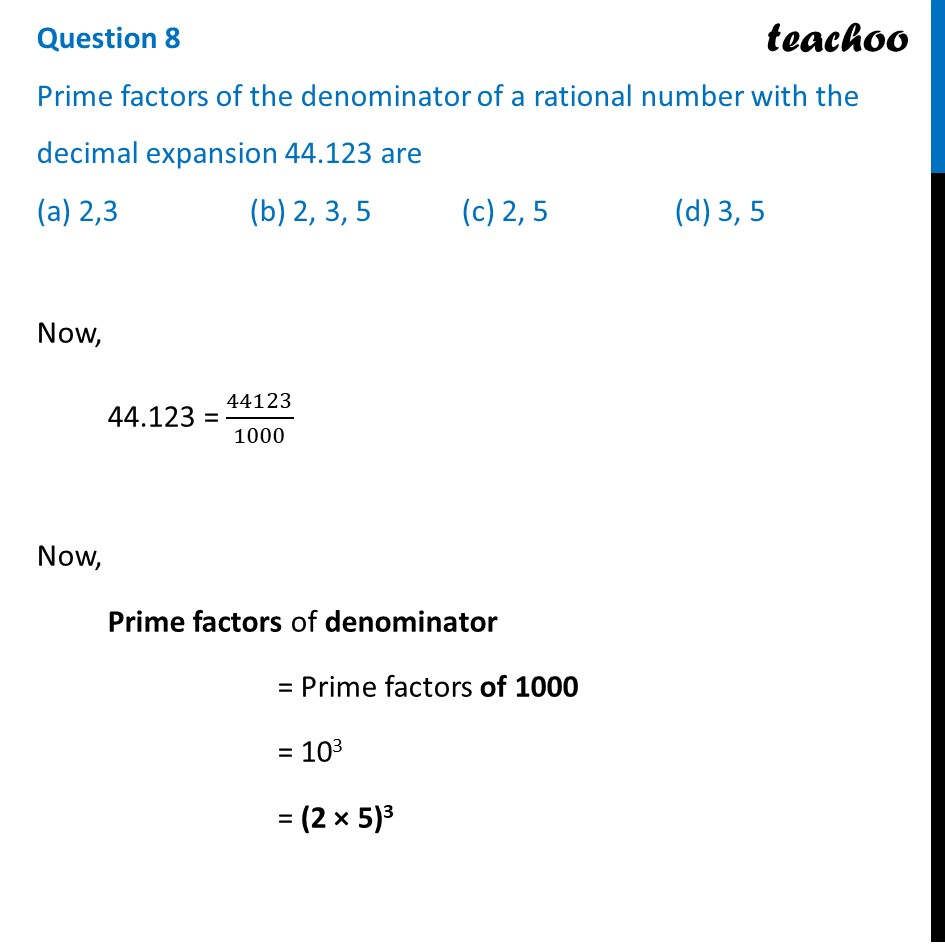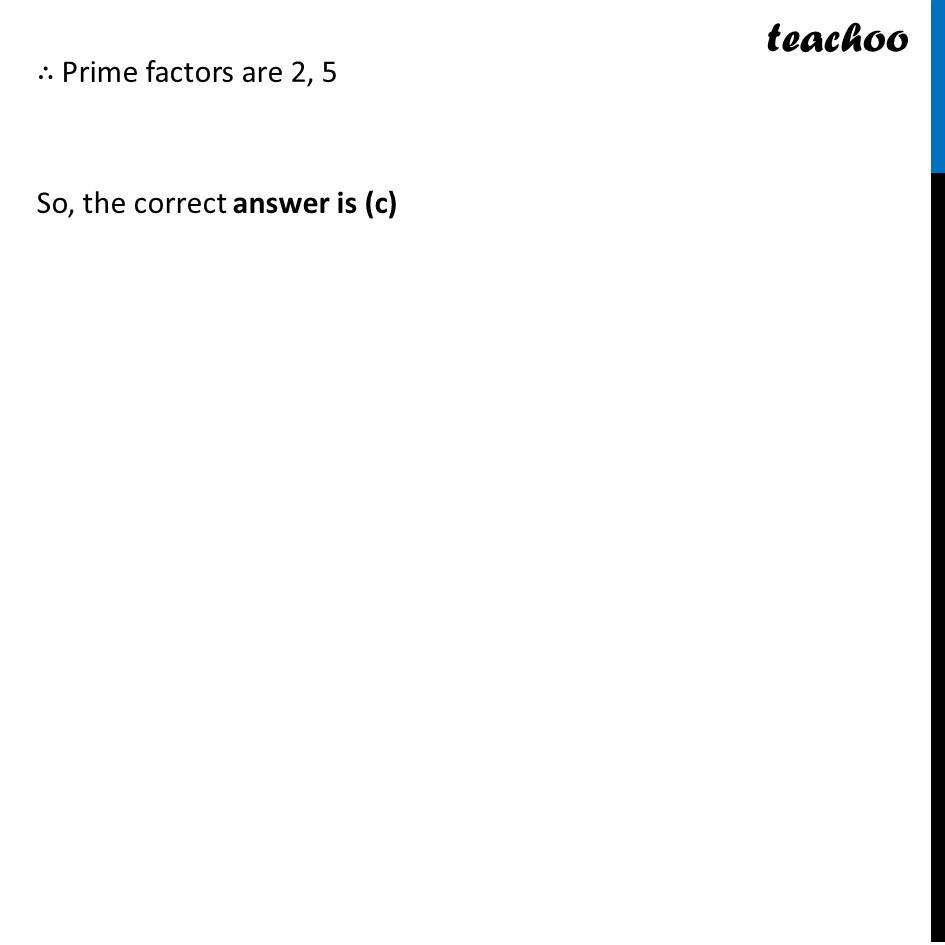## (a) 2,3   (b) 2, 3, 5   (c) 2, 5   (d) 3, 51. Class 10
2. Solutions of Sample Papers for Class 10 Boards
3. CBSE Class 10 Sample Paper for 2022 Boards - Maths Standard [MCQ]

Transcript

Question 8 Prime factors of the denominator of a rational number with the decimal expansion 44.123 are (a) 2,3 (b) 2, 3, 5 (c) 2, 5 (d) 3, 5 Now, 44.123 = 44123/1000 Now, Prime factors of denominator = Prime factors of 1000 = 103 = (2 × 5)3 ∴ Prime factors are 2, 5 So, the correct answer is (c)